# ggplot2: Themes

May 6, 2018
By

### Introduction

This is the last post in the series Elegant Data Visualization with ggplot2.
In the previous post, we learnt to combine multiple plots. In this post, we
will learn to modify the appearance of all non data components of the plot such
as:

• axis
• legend
• panel
• plot area
• background
• margin
• facets

### Libraries, Code & Data

We will use the following libraries in this post:

All the data sets used in this post can be found here and code can be downloaded from here.

### Basic Plot

We will continue with the scatter plot examining the relationship between
displacement and miles per gallon from the the mtcars data set.

``````p <- ggplot(mtcars) +
geom_point(aes(disp, mpg))
p``````### Axis

#### Text

The `axis.title.x` argument can be used to modify the appearance of the `X`
axis. In the below example, we modify the color and size of the title using
the `element_text()` function. Remember, whenever you are trying to modify the
appearance of a theme element which is a text, you must use `element_text()`.

You can use `axis.title.y` to modify the `Y` axis title and to modify the
title of both the axis together, use `axis.title`.

``p + theme(axis.title.x = element_text(color = "red", size = 10, face = "italic"))``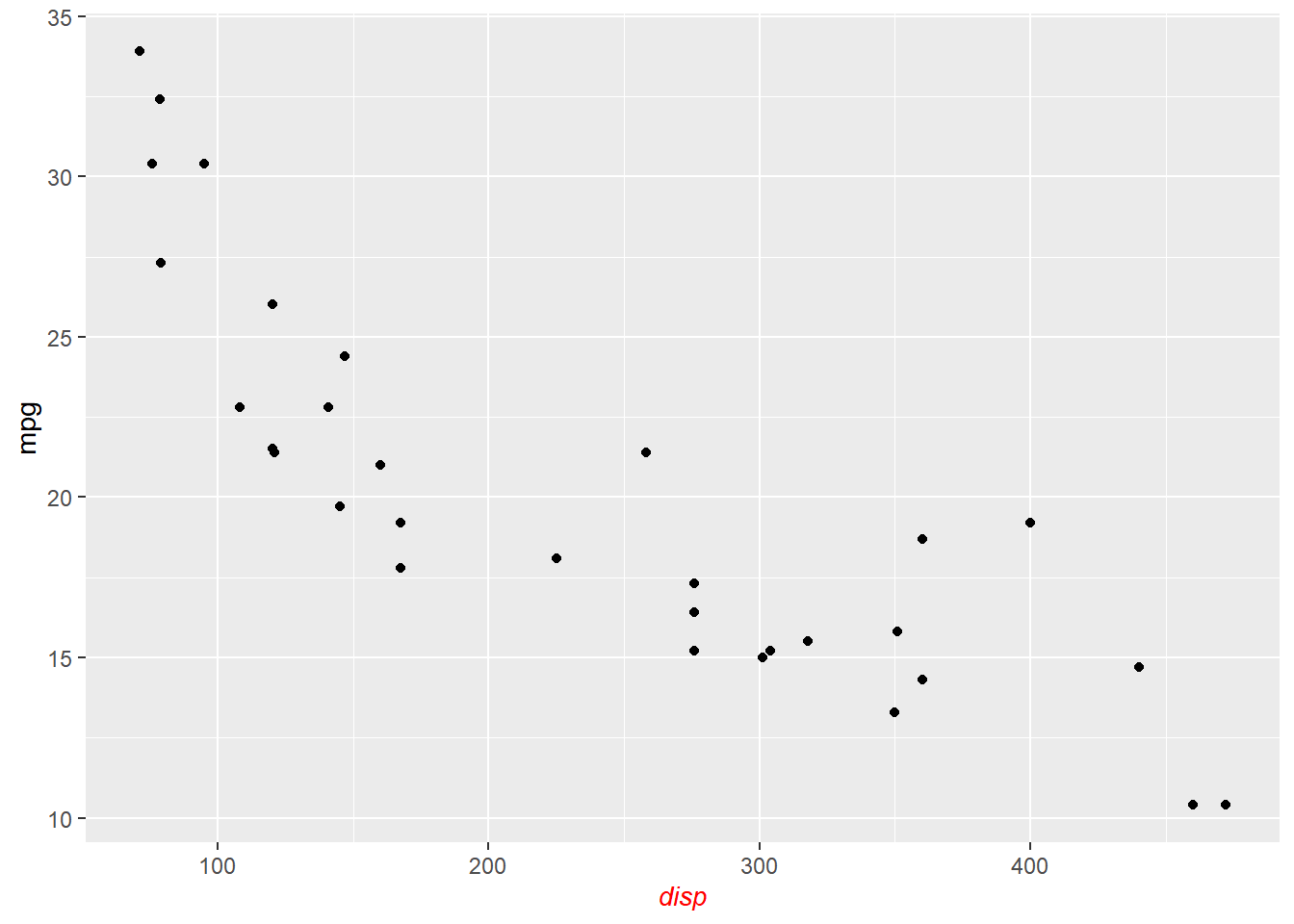#### Ticks

To modify the appearance of the axis ticks, use the `axis.ticks` argument. You can
change the color, size, linetype and length of the ticks using the `element_line()`
function as shown below.

``````p + theme(axis.ticks = element_line(color = 'blue', size = 1.25, linetype = 2),
axis.ticks.length = unit(1, "cm"))``````#### Line

The `axis.line` argument should be used to modify the appearance of the
axis lines. You can change the color, size and linetype of the line using
the `element_line()` function.

``p + theme(axis.line = element_line(color = 'red', size = 1.5, linetype = 3))``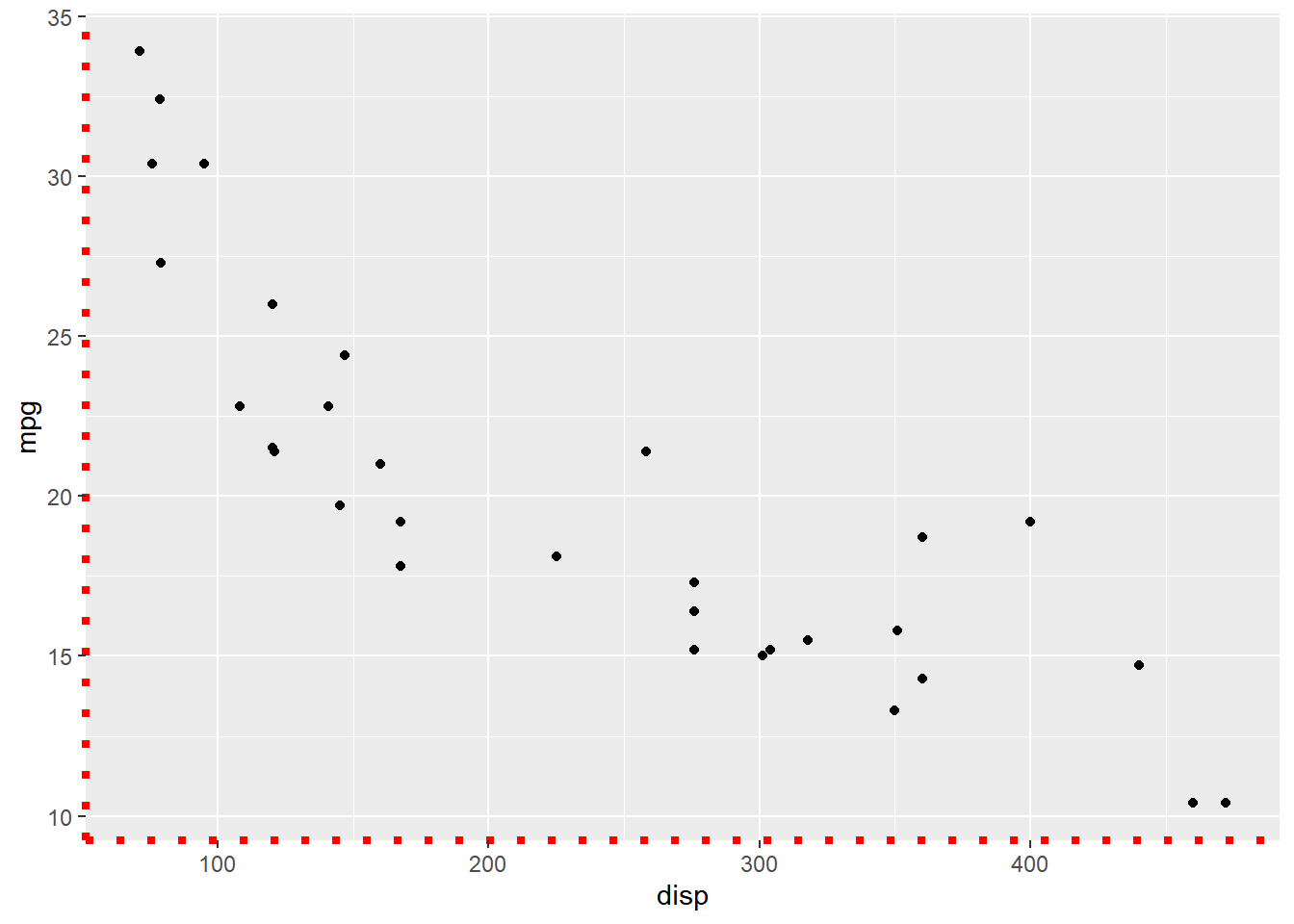### Legend

Now, let us look at modifying the non-data components of a legend.

``````p <- ggplot(mtcars) +
geom_point(aes(disp, mpg, color = factor(cyl), shape = factor(gear)))
p``````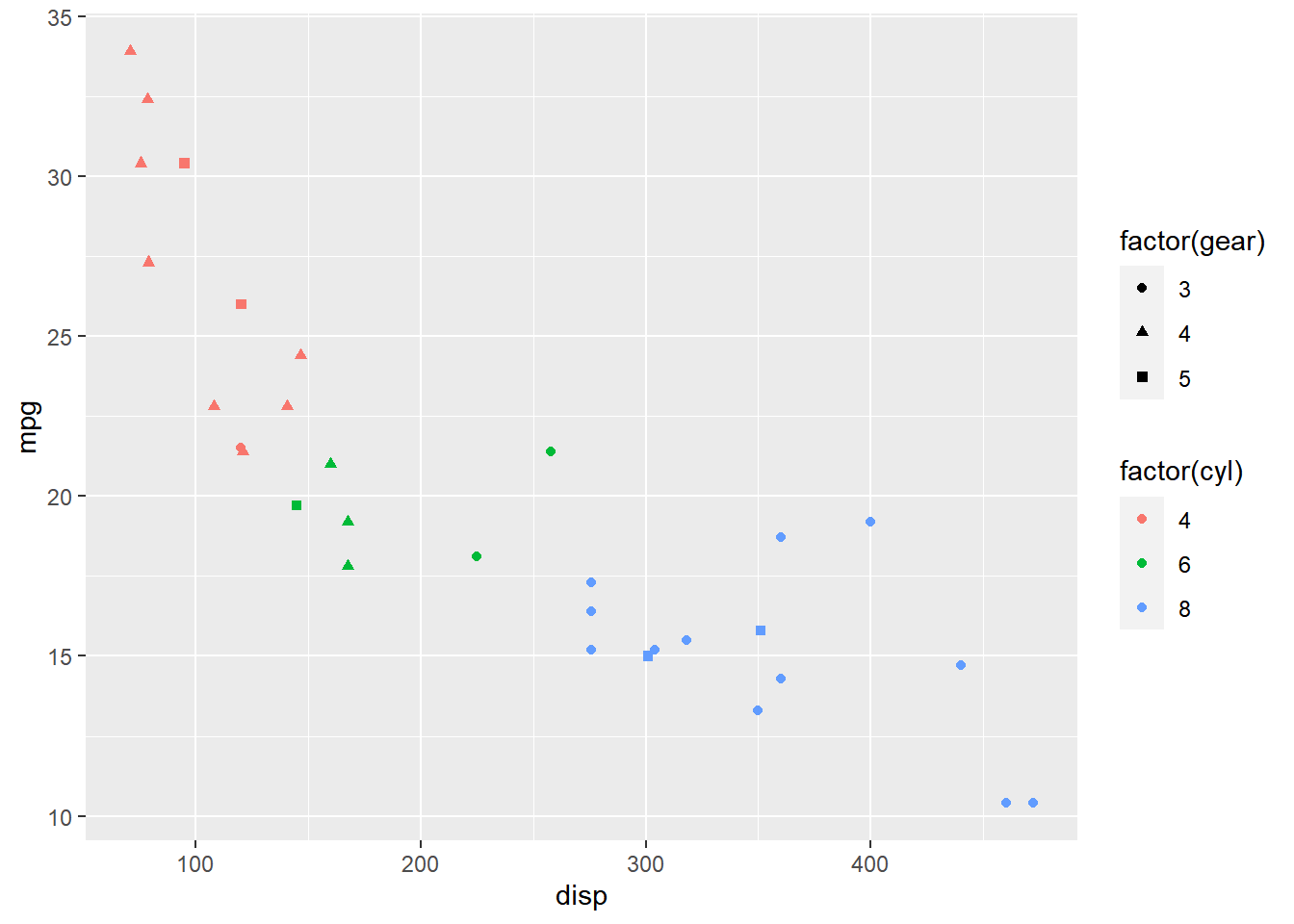#### Background

The background of the legend can be modified using the `legend.background`
argument. You can change the background color, the border color and line type
using `element_rect()`.

``````p + theme(legend.background = element_rect(fill = 'gray', linetype = 3,
color = "black"))``````#### Text

The appearance of the text can be modified using the `legend.text` argument.
You can change the color, size and font using the `element_text()` function.

``p + theme(legend.text = element_text(color = 'green', face = 'italic'))``#### Title

The appearance fo the title of the legend can be modified using the
`legend.title` argument. You can change the color, size, font and alignment
using `element_text()`.

``````p + theme(legend.title = element_text(color = 'blue', face = 'bold'),
legend.title.align = 0.1)``````#### Position

The position and direction of the legend can be changed using:

• `legend.position`
• and `legend.direction`
``p + theme(legend.position = "top", legend.direction = "horizontal")``### Themes

#### Classic Dark on Light

``````ggplot(mtcars) +
geom_point(aes(disp, mpg)) +
theme_bw()``````#### Default Gray

``````ggplot(mtcars) +
geom_point(aes(disp, mpg)) +
theme_gray()``````#### Light

``````ggplot(mtcars) +
geom_point(aes(disp, mpg)) +
theme_light()``````#### Minimal

``````ggplot(mtcars) +
geom_point(aes(disp, mpg)) +
theme_minimal()``````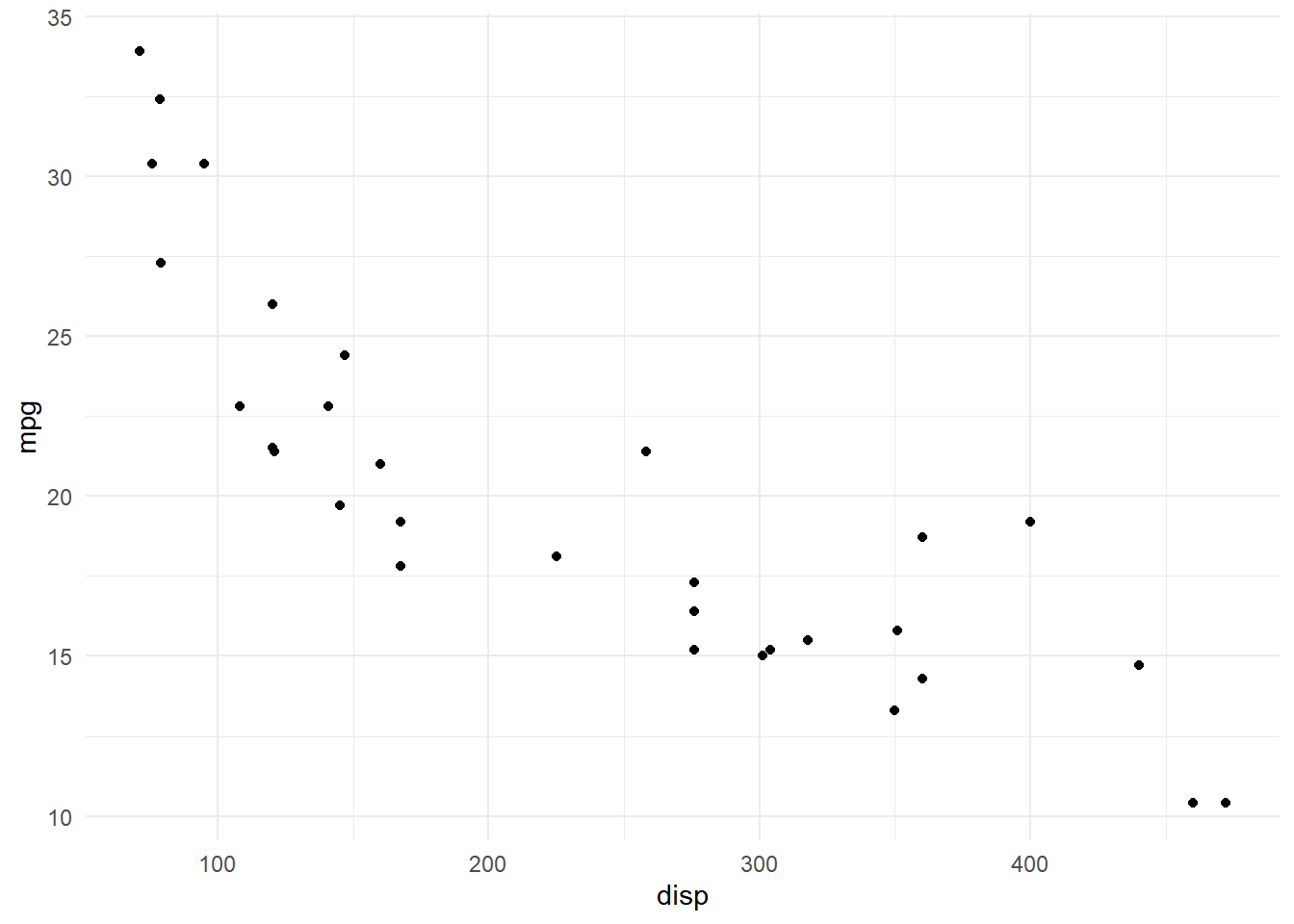#### Dark

``````ggplot(mtcars) +
geom_point(aes(disp, mpg)) +
theme_dark()``````#### Classic

``````ggplot(mtcars) +
geom_point(aes(disp, mpg)) +
theme_classic()``````#### Void (Empty)

``````ggplot(mtcars) +
geom_point(aes(disp, mpg)) +
theme_void()``````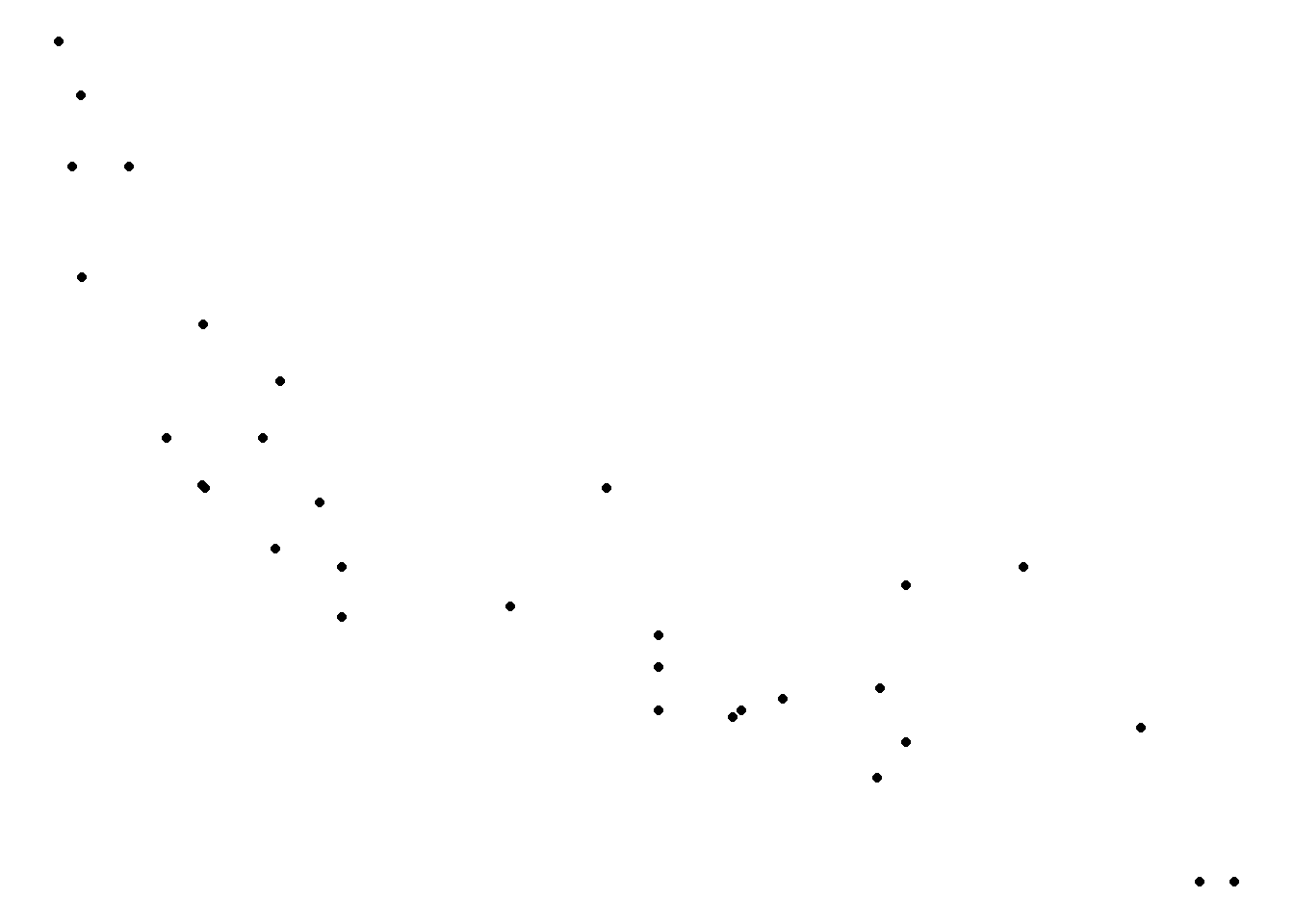### Summary

In this post, we learnt to modify the appearance of all non data components
of a plot and about the different themes available in ggplot2. This brings us
to the end of the series Elegant Data Visualization with ggplot2.

R-bloggers.com offers daily e-mail updates about R news and tutorials on topics such as: Data science, Big Data, R jobs, visualization (ggplot2, Boxplots, maps, animation), programming (RStudio, Sweave, LaTeX, SQL, Eclipse, git, hadoop, Web Scraping) statistics (regression, PCA, time series, trading) and more...